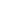科研动态最新资讯

• 天元讲堂（5.8）​From Bowen's eyes to physical measures in reparameterized linear toral flows with stopping points
• 浏览量：10 发布人： 发布时间：2020-05-14
• 题目：From Bowen's eyes to physical measures in reparameterized linear toral flows with stopping points

时间：2020/5/8 09:00-10:30

线上讲座，Zoom会议信息见下

报告摘要：Consider a constant vector field  $X = (1, \alpha)$ on $\mathbb{T}^2$. Multiply $X$ by a smooth function $f$ which is positive everywhere except at two points $p$ and $q$, where it vanishes. Let $\phi^t$ be the flow associated to this vector field. One can show that, for such a flow, the only invariant probability measures are combinations of Dirac measures at the points $p$ and $q$. A natural question arises: what happens to the time averages of $\phi^t(x)$ for a Lebesgue-typical point $x \in \mathbb{T}^2$ as $t$ tends to infinity? In this talk I will give some answers to that question.

This is a joint work with Pierre-Antoine Guihéneuf (Université Paris 6, France).

欢迎参加！

邀请人：动力系统与微分方程中心

Zoom会议信息：

会议时间：2020/5/15 09:00-10:30 https://us02web.zoom.us/j/3141591122?pwd=aWVGMGI2YkJQOXlEbk5IckRaRER2Zz09

会议 ID：314 159 1122

会议密码：123456